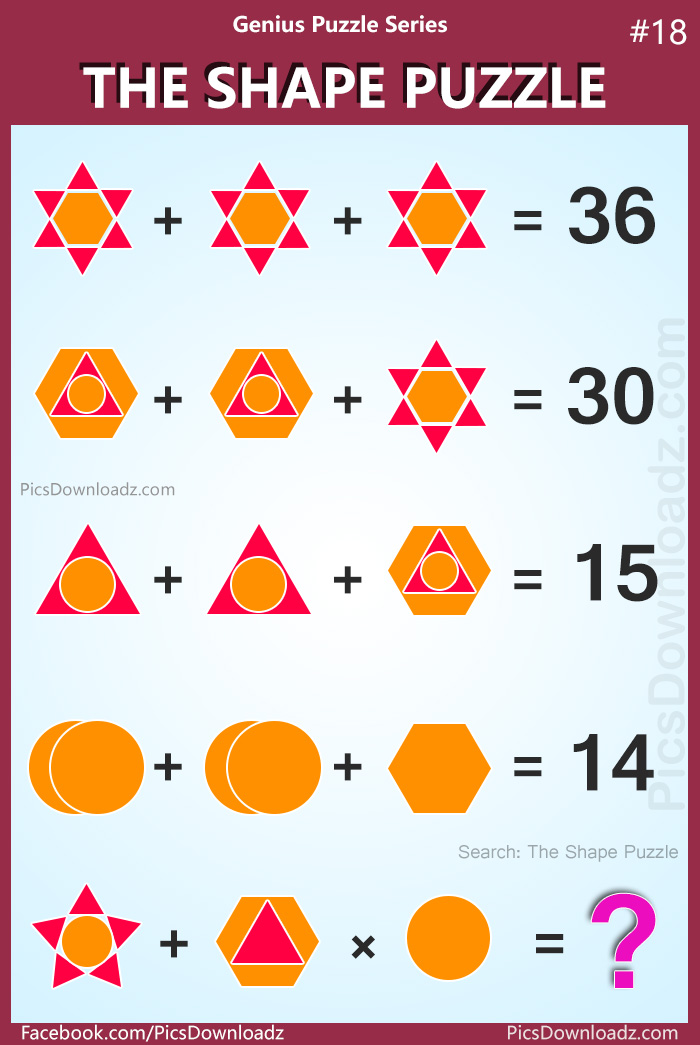This post may contain affiliate links. This means if you click on the link and purchase the item, We will receive an affiliate commission at no extra cost to you. See Our Affiliate Policy for more info.

The Shape Puzzle: Genius Puzzle Series #18 (With Answer)

0
41745

Best logical math puzzles; 90% will fail to answer this viral math puzzle. “The Shape Puzzle” Difficult Math Puzzles with the answer!

Welcome back to the Genius Puzzle Series. Another interesting puzzle is here “The Shape Puzzle”.

The shape’s used in this puzzle name as follows: Hexagon, Triangle, Circle.

Puzzle Question:

⇒ Star + Star + Star = 36

⇒ Hexagon + Hexagon + Star = 30

⇒ Triangle + Triangle + Hexagon = 15

⇒ Circle + Circle + Hexagon = 14

⇒ Star + Hexagon × Circle = ??

Let see, if you can solve this viral puzzle. Check out the image and share your equation or answer in the comment section below. Best of luck!

The Shape Puzzle: Genius Puzzle Series #18Join the Discussion here:

For those, who are not sure about the puzzle, here are some key points or hints:

• Each Shape has its own value, there’s a way to find it.
• Big & Small size does not affect the value of shapes

Want to print or share on your blog?

Simply download this puzzle image and print it out. Share this puzzle with your kids, students, and fellows. And for blog and website, you are free to use this image but you’re not allowed to edit the image and must attribute the image by linking to the original source, so we will be able to continue creating new puzzles & other amazing posts for you. Love <3

Solution:

Let us consider the shapes of these puzzle;

• Star* = One Hexagon with Six Triangle (in 1st eq.)
• Hexagon* = One Hexagon with Triangle and Circle in it. (in 2nd eq.)
• Triangle* = A Triangle with Circle in it. (in 3rd eq.)
• Circle* = Two Circles (in 4th eq.)

1st Equation;

⇒ Star* + Star* + Star* = 36

⇒ 3 Star* = 36

⇒ Star* = 36/3

Star* = 12

2nd Equation;

⇒ Hexagon* + Hexagon* + Star* = 30

⇒ 2 Hexagon* + Star* = 30

⇒ 2 Hexagon* + 12 = 30

⇒ 2 Hexagon* = 30 – 12

⇒ Hexagon* = 18/2 = 9

Hexagon* = 9

3rd Equation;

⇒ Triangle* + Triangle* + Hexagon* = 15

⇒ 2 Triangle* + 9 = 15

⇒ 2 Triangle* = 15 – 9

⇒ Triangle* = 6/2 = 3

Triangle* = 3

4th Equation;

⇒ Circle* + Circle* + Hexagon = 14

⇒  2 Circle* + Hexagon = 14

We know:

• Triangle* = 3 (from 3rd eq.)
• Hexagon* = 9 (from 2nd eq.)

Lets find the simple Hexagon, 3rd equation;

• Hexagon = Hexagon* – Triangle*
• Hexagon = 9 – 3
• Hexagon = 6

Now put the Hexagon value in eq. 4th

⇒  2 Circle* + Hexagon = 14

⇒  2 Circle* + 6 = 14

⇒  2 Circle* = 14 – 6

⇒  Circle* = 8/2 = 4

⇒  Circle* = 4

⇒  Circle* = 4 Then Single Circle = 2

5th or Last Equation;

⇒ Star** + Hexagon** × Circle = ??

Lets focus on the details of this equation;

• Star** = One Circle with 5 Triangle
• Hexagon** = One Hexagon with One Triangle in it
• Circle = Just a simple circle

Now we already know each shape value separately, i.e.

• Hexagon = 6
• Circle = 2
• Triangle* = 3, (Triangle with a circle in it)
• Simple Triangle = Triangle* – Circle = 3 – 2 = 1

Now, rewrite the 5th eq.

⇒ Star** + Hexagon** × Circle = ??

⇒ (One Circle with Five Triangle) + ( One Hexagon with a triangle) × (One Circle) = ??

⇒ (2+1+1+1+1+1) + (6+1) × (2) = ??

⇒ (7) + (7) × (2) = ??

⇒ 7 + 14 = 21 Ans

Search items:

The Shape Puzzle, Genius Puzzle Series 18, Shape Puzzle 18, Viral Shape Math Puzzle, Hard Math Puzzles Images, Facebook Math Puzzle Image, Viral Whatsapp Image Puzzle, Star Star Star 36, Hexagon Triangle Circle Puzzle, Puzzles for Adults Logical Puzzle Question, Only for Genius Puzzles, Facebook Shape Puzzle, Hexagon Star Puzzle, the Shape puzzle answer.